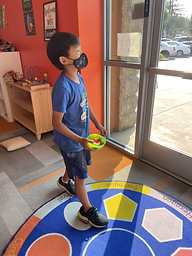## Ms. Caitlyn

### Target 1​

###### Lesson Type:

New

Data Probability

:

Ratio

Understand ratio concepts and use ratio reasoning to solve problems.

###### 1:

Understand that a ratio represents a comparison of two quantities.

6th

###### Vocabulary:

Ratio, Comparison, Quantities

Activities:

Students went on a scavenger hunt for six different types of fruit.

Students created a table to show the quantities of each fruit.

Students wrote the ratio of one fruit to another both numerically and in words (ex.: 6 oranges and 3 apples are notated as oranges : apples or 6 : 3).### Home Exploration

###### Guiding Questions:## Absent Students:

### Target 2

:

###### 1:

Identify in a given problem what the unknown information is.

###### 2:

Read, write, and evaluate expressions in which letters stand for numbers.

6th

###### Vocabulary:

Variable, Unknown, Expression

Activities:

Students solved puzzles where each symbol represented an unknown number.

Students connected the puzzles to algebraic expressions.### Home Exploration

###### Guiding Questions:### Target 3

:

###### 1:

Solve problems that have more than one operation.

###### 2:

Understand that the presence of parenthesis in an arithmetic equation signals for this part of the problem to be solved first.

###### 3:

Understand that the order of operations must be followed to evaluate expressions.

###### 4:

Define PEMDAS – Parenthesis, Exponents, Multiplication, Division, Addition, Subtraction.

###### 5:

Correctly identify the order of operations and use them to simplify expressions.

5th

###### Vocabulary:

Order of Operations, PEMDAS, Parentheses

Activities:

Students solved multi-step problems that were written on Post-Its.

Students were given ten Post-Its each and raced to see who could solve the most problems quickly and accurately.### Home Exploration